# Question to be investigated

 A3- HALL EFFECT Last Revision: August, 21 2007 QUESTION TO BE INVESTIGATED How to individual charge carriers behave in an external magnetic field that is perpendicular to their motion? INTRODUCTION The Hall effect is observed when a magnetic field is applied at right angles to a rectangular sample of material carrying an electric current. A voltage appears across the sample that is due to an electric field that is at right angles to both the current and the applied magnetic field. The Hall effect can be easily understood by looking at the Lorentz force on the current carrying electrons. The orientation of the fields and the sample are shown in Figure 1. An external voltage is applied to the crystal and creates an internal electric field (Ex). The electric field that causes the carriers to move through the conductive sample is called the drift field and is in the x-direction in Figure 1. The resultant drift current (Jx) flows in the x-direction in response to the drift field. The carriers move with an average velocity given by the balance between the force accelerating the charge and the viscous friction produced by the collisions (electrical resistance). The drift velocity appears in the cross product term of the Lorentz force as shown in equation 1. The transverse (y) component of the Lorentz force causes charge densities to accumulate on the transverse surfaces of the sample. Therefore, an electric field in the y-direction results that just balances the Lorentz force because there is no continuous current in the y-direction (Only a transient as the charge densities accumulate on the surface). The equilibrium potential difference between the transverse sides of the sample is called the Hall voltage.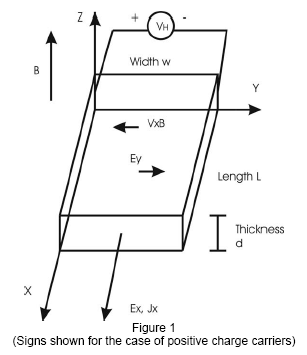A good measurement of the Hall voltage requires that there be no current in the y-direction. This means that the transverse voltage must be measured under a condition termed “no load”. In the laboratory you can approximate the “no load” condition by using a very high input resistance voltmeter. THEORY The vector Lorentz force is given by: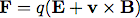(1) where F is the force on the carriers of current, q is the charge of the current carriers, E is the electric field acting on the carriers, and B is the magnetic field inside the sample. The charge may be positive or negative depending on the material (conduction via electrons or “holes”). The applied electric field E is chosen to be in the x-direction. The motion of the carriers is specified by the drift velocity v. The magnetic field is chosen to be in the z-direction (Fig. 1). The drift velocity is the result of the action of the electric field in the x-direction. The total current is the product of the current density and the sample’s transverse area A (I = JxA; A = wt). The drift current Jx is given by;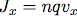(2) where n is the number density or concentration of carriers. The carrier density n is typically only a small fraction of the total density of electrons in the material. From your measurement of the Hall effect, you will measure the carrier density. In the y-direction assuming a no load condition the free charges will move under the influence of the magnetic field to the boundaries creating an electric field in the y-direction that is sufficient to balance the magnetic force.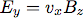(3) The Hall voltage is the integral of the Hall field (Ey=EH) across the sample width w.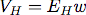(4) In terms of the magnetic field and the current: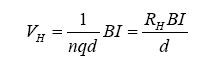(5) Here RH is called the Hall coefficient. Your first task is to measure the Hall coefficient for your sample. This equation is where you will begin. The measurements you will take will be of VH as a function of drift current I and as a function of magnetic field B. You will need to fix one parameter in order to intelligibly observe VH. This experiment is setup to measure VH as a function of I, so you will fix B for each trial you perform. Other important and related parameters will also be determined in your experiment. The mobility μ is the magnitude of the carrier drift velocity per unit electric field and is defined by the relation: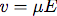(6) or,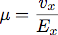(7) This quantity can appear in the expression for the current density and its practical form.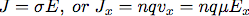(8) We have the relations: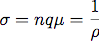(9) Where σ is the conductivity and ρ is the resistivity. The total sample resistance to the drift current is: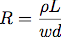(10) Not only does the Hall coefficient give the concentration of carriers it gives the sign of their electric charge, by: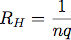(11) The dimensions of the sample are w, the width which is to be oriented in the y-direction, d the thickness which is to be oriented in the z-direction which is perpendicular to the magnetic field and L, the length of the sample which is to be located along the x-direction. The sample should be about four times longer than it is wide so that the electric current streamlines have an opportunity to become laminar or the electric potential lines to become parallel and perpendicular to the edges of the sample. The Hall voltage should be zero when the sample is not in a magnetic field and the drift current is applied. A plot of Hall voltage as a function of drift current at constant magnetic field will have a slope equal to RHB/d. Thus, the slope multiplied by d/B is the magnitude of the Hall coefficient. Pay careful attention to the direction of fields and the sign of the voltages and obtain the sign of the charge carriers. For some materials the Hall coefficient is reasonably constant in the above equation and not a function of any of the experimental parameters. For some materials the Hall constant is a function of the magnetic field due to a magnetoresistance effect. Nevertheless, the Hall voltage is directly related to the magnetic field and the drift current, and it is inversely related to the thickness of the sample. The samples used for the measurement are made as thin as possible to produce the largest possible voltage for easy detection. The sample has no strength to resist bending and the probe is to be treated with great care. This applies to the probe of the Gaussmeter as well. Do not attempt to measure the thickness of the sample. The values for the dimensions of your sample are: w = 0.152 cm, width, L = 0.381 cm, length, d = 0.0152 cm, thickness. The Hall effect probe is a thin slab of indium arsenide, InAs, cemented to a piece of fiberglass. A four lead cable is attached so that the necessary electrical circuit can be used to detect the Hall voltage. The probe is equipped with a bakelite handle that is used to hold the probe in place. The white and green wires are used to measure the Hall voltage and the red and black wires carry the drift current. EXPERIMENT Before connecting the circuit, carefully measure the resistance of the current limiting resistor with an ohm meter (the resistance should be close to 100 ohms). Connect the probe into the electrical circuit shown in Figure 2 (See Appendix). You are to measure the Hall voltage, the drift current (determined from the voltage across the current limiting resistor and the known voltage across the system), and the drift voltage (this is the applied voltage minus voltage across the current limiting resistor). You have to keep track of directions and vectors. Before you turn on the power, please make the following checks: Check the circuit carefully to ensure it makes sense to you. Put the meters on their correct scales. The voltage across the current limiting resistor will be less than 1 Volt. The Hall voltage will be even less. As the drift current is stepped up by the computer, check the following: The drift current must not exceed 10ma this corresponds to 1.0 volt across the 100 ohm current limiting resistor (This voltage is shown on one of the voltmeters in Figure 2). Download 318 Kb.Do'stlaringiz bilan baham: